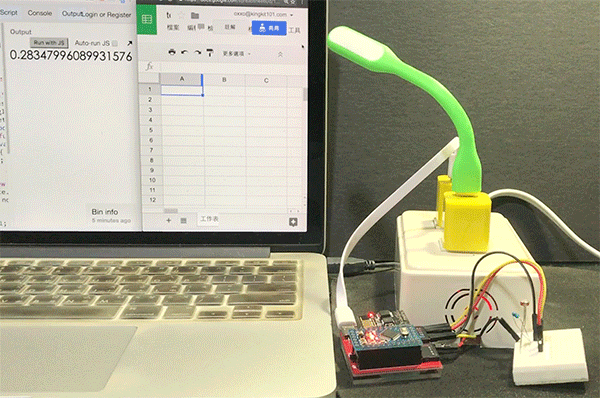Webduino 學習手冊網站即將改版，提供您更好的閱讀體驗！

# Google 試算表 ( 原理 )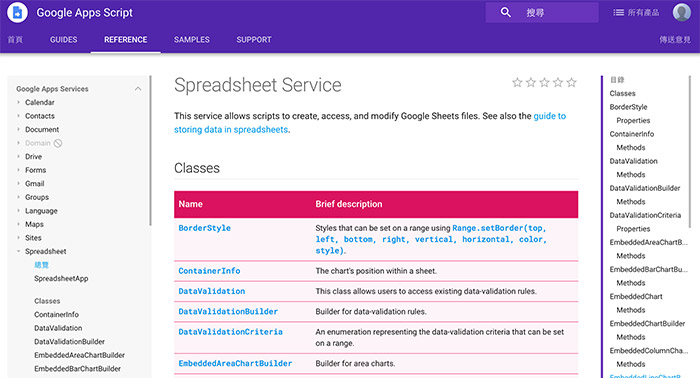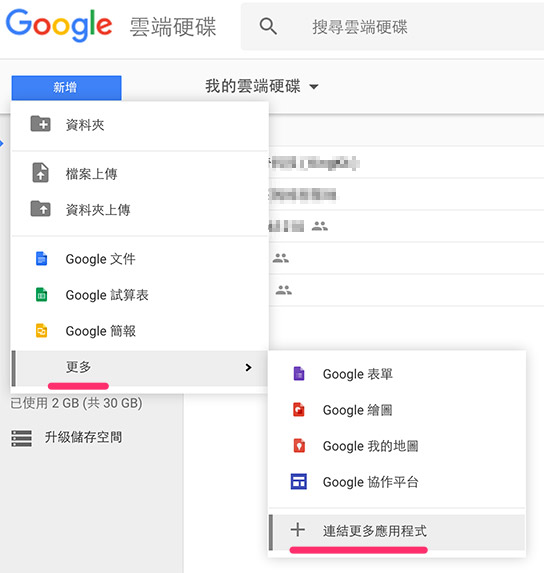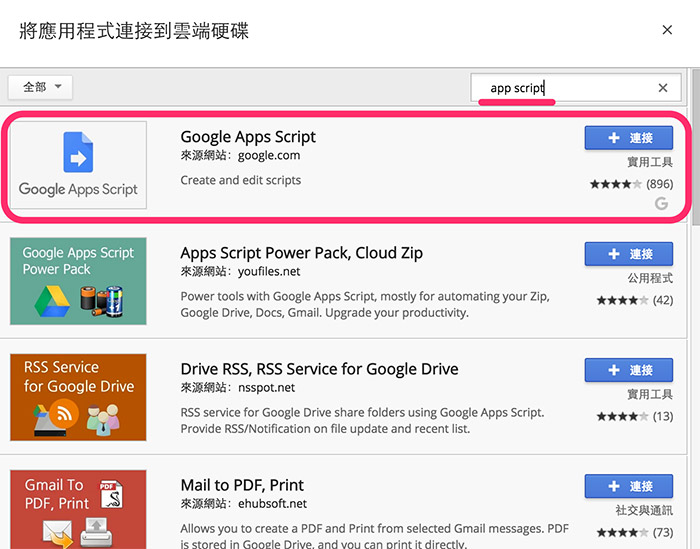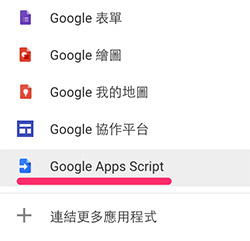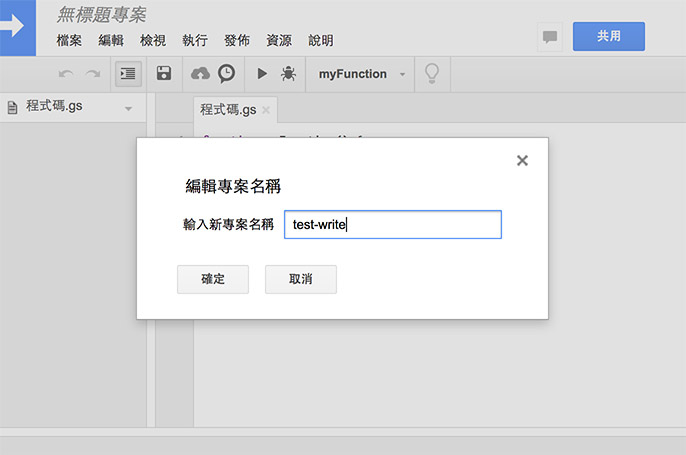``````function doGet(e) {
}
``````

``````function doGet(e) {
var params = e.parameter;
var data = params.data;
}
``````

``````var sheetUrl = params.sheetUrl;
var sheetTag = params.sheetTag;

``````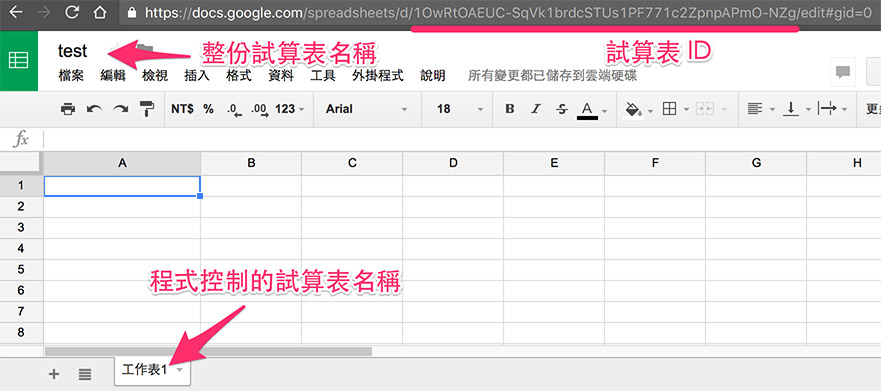`getLastRow` 的方法是讓我們可以獲取最後一行 ( 如果你的資料有 100 行，最後一行就是 100 )，這樣我們儲存資料就可以放在 `LastRow+1` 行。

``````var LastRow = Sheet.getLastRow();
``````

``````//寫入資料
data = data.split(',');
data.forEach(function(e,i){
Sheet.getRange(LastRow+1, i+1).setValue(e);
});

//回傳處理完成
return ContentService.createTextOutput(true);
``````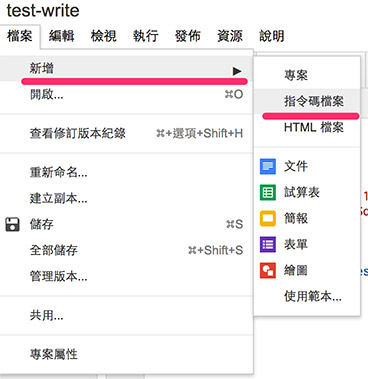``````function debug() {
var date = new Date();
var now = date.getHours() + ':' + date.getMinutes() + ':' + date.getSeconds();
doGet(
{
parameter:{
data: now+',oxxo,123',
sheetUrl:"試算表網址",
sheetTag:"工作表1"
}
}
);
}
``````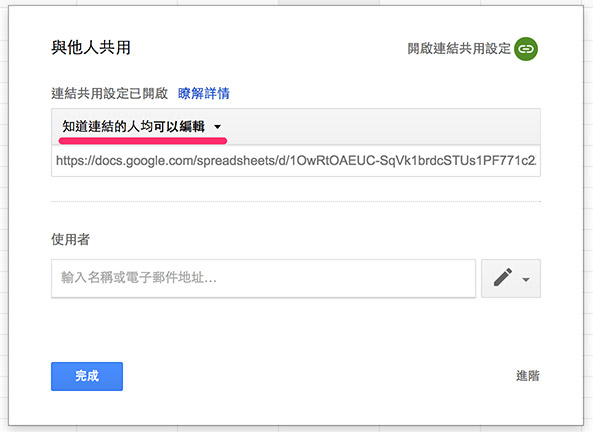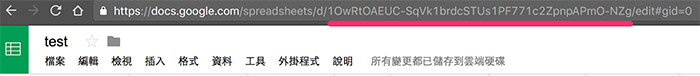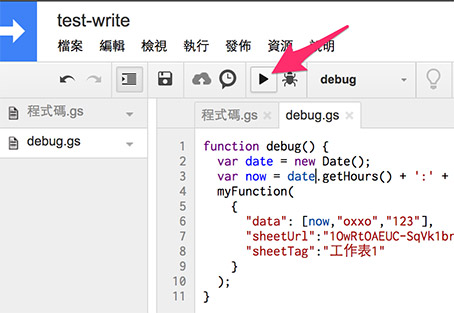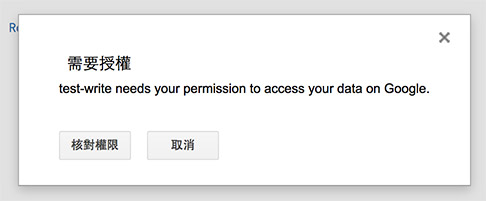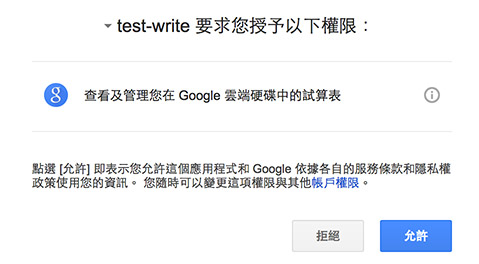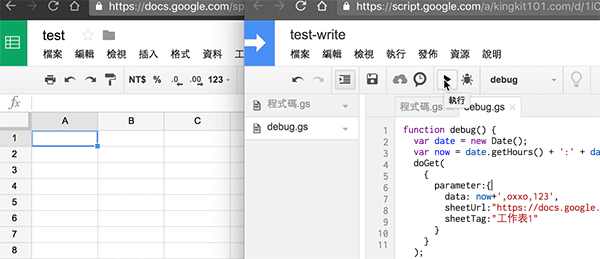``````function doGet(e) {
var params = e.parameter;
var data = params.data;
var sheetUrl = params.sheetUrl;
var sheetTag = params.sheetTag;

//插入第一行空白
Sheet.insertRowBefore(1);

//寫入資料
data = data.split(',');
data.forEach(function(e,i){
Sheet.getRange(1, i+1).setValue(e);
});

return ContentService.createTextOutput(true);
}
``````

## 部署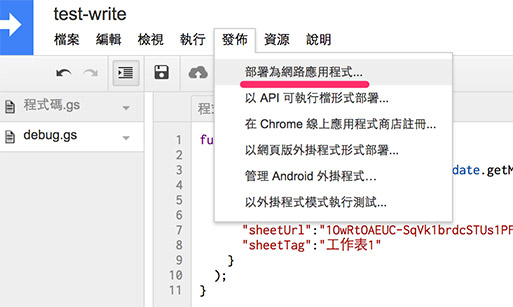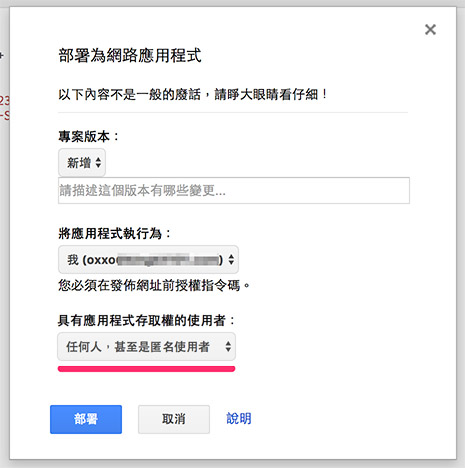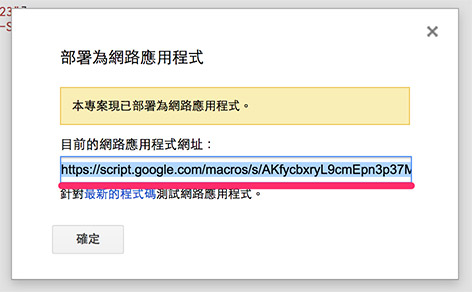## 透過網頁寫入資料

``````<!doctype html>
<html>
<meta charset="utf-8">
<title></title>

<body>
第一欄：<input id="a1"><br/>
第二欄：<input id="a2"><br/>
第三欄：<input id="a3"><br/>
<button id="b">儲存</button>
</body>
</html>
``````

JavaScript 會透過 jQuery 的 `get` 來寫入資料，基本上程式碼和剛剛的 debug 差不多。

``````\$(function() {
var \$a1 = \$('#a1'),
\$a2 = \$('#a2'),
\$a3 = \$('#a3'),
\$b = \$('#b'),
a = {};

\$b.on('click', function() {
a = {
data: \$a1.val()+','+ \$a2.val()+','+\$a3.val(),
sheetUrl: '你的試算表網址',
sheetTag: '工作表1'
};
console.log(a);
});
});
``````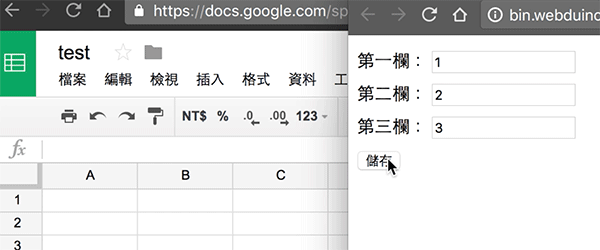## 儲存開關燈時間資訊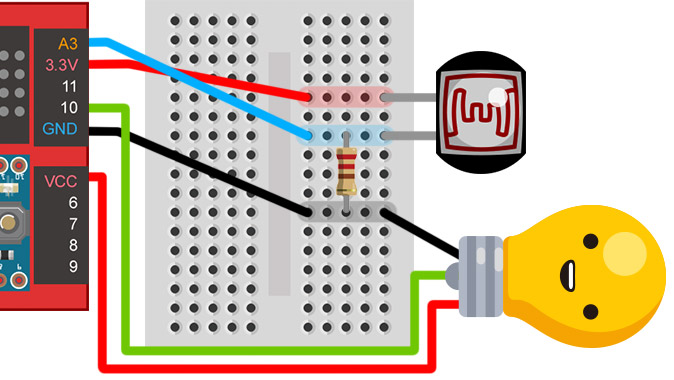JavaScript 的部分除了光敏電阻、LED 與剛剛的試算表程式宣告外，就是多了兩個變數，這兩個變數的目的在於避免試算表隨時都在記錄燈泡資訊 ( 因為類比訊號由 `board.samplingInterval` 設定，目前為 0.25 秒偵測一次 )，所以透過這兩個變數的判斷，可以只在開燈或關燈的時候紀錄。

``````var photocell , led , date , now;
var a = {};
var b = 0;
var c = 0;
var \$show = \$("#show");

board.systemReset();
board.samplingInterval = 250;
photocell = getPhotocell(board, 3);
led = getLed(board, 10);
photocell.on(function(val){
\$show.text(val);
if(val<0.2){
b = b + 1;
c = 0;
led.on();
date = new Date();
now = date.getHours() + ':' + date.getMinutes() + ':' + date.getSeconds();
a.data = now+',"打開",'+val;
}else{
b = 0;
c = c + 1;
led.off();
date = new Date();
now = date.getHours() + ':' + date.getMinutes() + ':' + date.getSeconds();
a.data = now+',"關起來",'+val;
}
if(b==1||c==1){
a.sheetUrl = '你的試算表網址';
a.sheetTag = '工作表1';
}
});
});
``````